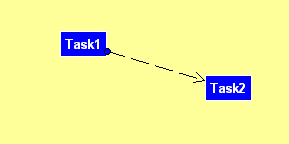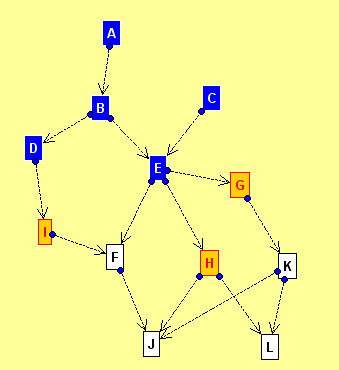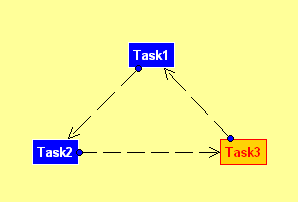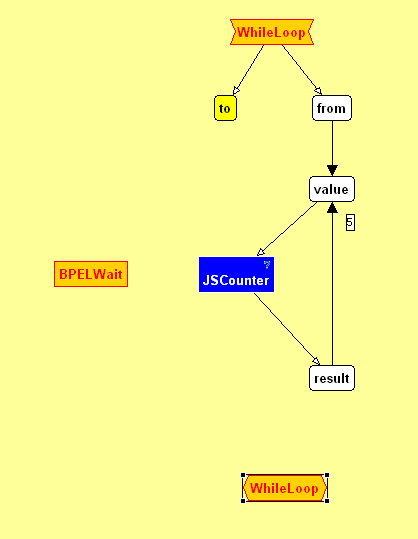## 9.1: Basic Patterns

### 9.1.1: Empty Process

Empty processes are supported. You can use them to perform a quick mapping between their input and output parameters.

### 9.1.2: Sequential

Sequential execution of tasks is achieved by linking tasks in the control flow sequentially, as depicted in Figure 9.1.In the case depicted in the previous figure, Task1 is executed before Task2. This control flow dependency is expressed as follows in the activator and condition properties of the tasks:

The activator of Task1 is left empty meaning that it will be invoked as soon as the process execution is started. Task2 on the other hand has an activator which expresses the dependency on Task1:
```Finished(Task1)
```

### 9.1.3: Parallel

Parallel execution of two or more tasks is achieved by identically setting their activators to express their dependency on the previous task or on the start of the process.In the case depicted in Figure 9.2, execution of tasks Task2a and Task2b are supposed to happen in parallel after Task1 has been executed. Their activators are thus both set to "Finished(Task1)":

If both tasks were supposed to be executed in parallel upon start of the process (without depending on Task1) the activators of both would be left empty meaning that both tasks are to be executed when the process starts executing.

### 9.1.4: Flow

In general, any Directed Graph can be used to specify arbitrary dependencies between a set of tasks.

Note: You can also work with cyclic graphs, as long as you update some of the activators on the merging points not to introduce deadlocks (See 9.4: Loops for more information on how to do this).Figure 9.3: Arbitrary Flow task execution

Dependencies can be drawn on the Control Flow view (Figure 9.3). JOpera will automatically translate them into a set of activator expressions, like in the following:

ActivityATRUE
ActivityBFinished(A)TRUE
ActivityCTRUE
ActivityDFinished(B)TRUE
ActivityEFinished(C) AND Finished(B)TRUE
ActivityFFinished(E) AND Finished(I)TRUE
ActivityGFinished(E)TRUE
ActivityHFinished(E)TRUE
ActivityIFinished(D)TRUE
ActivityJFinished(F) AND Finished(H) AND Finished(K)TRUE
ActivityKFinished(G)TRUE
ActivityLFinished(H) AND Finished(K)TRUE

Note: You can also manually change the activator by selecting a task and editing the corresponding property

## 9.2: Branching Control Flow Patterns

### 9.2.1: Parallel Split

A point in the process where a set of activities can be executed in any order (even in parallel).

ActivityATRUE
ActivityB1Finished(A)TRUE
ActivityB2Finished(A)TRUE

Since the activators of both B1 and B2 fire when task A is finished, they will be both executed in parallel.

### 9.2.2: Synchronization

A point in the process where multiple independent paths (A, B) must be synchronized before execution continues with C

ActivityATRUE
ActivityBTRUE
ActivityCFinished(A) AND Finished(B)TRUE

### 9.2.3: Simple Merge

A point in the process where two or more exclusively alternative branches come together without synchronization. Use the choice process parameter (A, B) to control which of the two initial tasks (A xor B) will be executed.

ActivityA(PROC.choice = "A")
ActivityB(PROC.choice = "B")
ActivityCFinished(A) OR Finished(B)TRUE

Since by definition only A xor B can run, the activator in C can be safely set to Finished(A) OR Finished(B). For scenarios where this assumption does not hold (A or B) have a look at the MultiMerge and the Discriminator examples.

### 9.2.4: Exclusive Choice

A point in the process, where one out of several branches is chosen.

ActivityATRUE
ActivityBFinished(A)PROC.choice = "B"
ActivityCFinished(A)PROC.choice = "C"

To choose which task (B or C) is executed set the choice input parameter of the process to the corresponding task name. Look at the conditions associated with the two tasks to see how this works.

### 9.2.5: Multiple Choice

A point in the process, where a number of branches can be chosen.

ActivityBFinished(A)(PROC.choice = "B") OR (PROC.choice = "BC")
ActivityCFinished(A)(PROC.choice = "C") OR (PROC.choice = "BC")
ActivityATRUE

Considering the conditions associated with the B and C tasks, it is possible to run either B, C or both by setting the appropriate value (B, C, BC) into the choice input parameter of the process.

### 9.2.6: Synchronizing Merge

This process will synchronize at task D, two alternative paths (task B or task C) that can both be taken at the same time, but also taken as alternatives. To control which of B or C (or both) is taken, set the choice process input parameter to one of the following values (B, C, BC).

ActivityATRUE
ActivityBFinished(A)(PROC.choice = "B") OR (PROC.choice = "BC")
ActivityCFinished(A)(PROC.choice = "C") OR (PROC.choice = "BC")
ActivityD((Finished(B) AND Unreachable(C)) OR (Finished(C) AND Unreachable(B)) OR (Finished(B) AND Finished(C)))TRUE

### 9.2.7: Multiple Merge

Branches converge without synchronization. If more than one branch gets activated, possibly concurrently, the activity following the merge is started for every activation of every incoming branch. This is exactly the semantics of the "OR" in the activator associated with task D.

ActivityATRUE
ActivityBFinished(A)(PROC.choice = "B") OR (PROC.choice = "BC")
ActivityCFinished(A)(PROC.choice = "C") OR (PROC.choice = "BC")
ActivityD(Finished(B) OR Finished(C))TRUE

### 9.2.8: N out of M Join

This example shows how to merge 2 out of 3 paths. The execution of D will take place if any pair out of the triplet (task A, task B, task C) has finished.

ActivityATRUE
ActivityBTRUE
ActivityCTRUE
ActivityD(Finished(A) AND Finished(B)) XOR (Finished(B) AND Finished(C)) XOR (Finished(A) AND Finished(C))TRUE

## 9.3: Conditional Execution

Every JOpera tasks is associated with a condition. This is a boolean expression, which is evaluated based on the content of the data flow parameters. If it is satisfied, the task is started. Otherwise the task execution is skipped. By default all conditions are set to `TRUE``, so tasks are always executed as soon as their control flow activator is triggered.` In this section we give some examples of conditions to explain how they can be set. Conditions of different kinds can be mixed using the usual binary boolean operators (AND, OR, XOR). Look at the `conditions.oml` example file shipped with JOpera for additional examples.

### 9.3.1: Simple Local Task Input Conditions

These are the simplest conditions that refer to the input parameters of the task to which the condition is associated with.

```Parameter = Value
```
The `Parameter` is one of the input parameters of the task the condition is associated with.
• ```A = B
```
Start the task if the values of its input parameters `A` and `B` are equal.
• ```p <> "XYZ"
```
Start the task if the value of its input parameters `p` is not equal to the string "XYZ".

These conditions refer to the values of the output parameters of tasks. They should refer to tasks that come before the current one in the order of execution (control flow).

```Task.Parameter = Value
```
The `Parameter` is one of the output parameters of the task called `Task`.
• ```T.A = "X"
```
The task will start if the output parameter `A` of task `T` is equal to the string value "X".
• ```T1.A = T2.B
```
The task will start if the output parameter `A` of task `T1` is equal to the output parameter `B` of task `T2`.

### 9.3.3: Process Input Conditions

To refer to the input parameters of a process, use the `PROC` identifier.

```PROC.p = "X"
```
The task will start if the input parameter `p` of the process is equal to the string valuel "X"
• ```T.A <> PROC.p
```
The task will start if the output parameter `A` of task `T` is not equal to the process input parameter called `p`

### 9.3.4: General Conditions

These conditions can refer to both input or output parameters of tasks. Use the `IN` or `OUT` prefix to refer to the corresponding input or output parameters. If no prefix is specified, then the default is to refer to the output parameters.

```Task.[IN|OUT].Parameter = Value
```
Examples:
• ```T1.IN.a = null
```
The task will start if the input parameter `a` of task `T1` is null
• ```T1.IN.a = T2.IN.b
```
The task will start if the input parameter `a` of task `T1` is equal to the value of the input parameter `b` of task `T2`
• ```T1.IN.a = T2.OUT.b
```
The task will start if the input parameter `a` of task `T1` is equal to the value of the output parameter `b` of task `T2`
• ```T1.IN.a = T2.b
```
The task will start if the input parameter `a` of task `T1` is equal to the value of the output parameter `b` of task `T2`

### 9.3.5: System Parameter Conditions

It is also possible to write conditions that also refer to system input or output parameters of tasks. Use the `SYS``, ``SYSIN` or `SYSOUT` prefix to refer to the corresponding system input or output parameters.

```Task.[SYS|SYSIN|SYSOUT].Parameter = Value
```

### 9.3.6: General Syntax for Conditions

Since version 2.4.8, JOpera supports the following syntax for referring to data flow parameter values from the conditions expressions associated with each task.

```[PROC|TaskName].[IN|OUT|SYS|SYSIN|SYSOUT].ParameterName =|<>|<|> Value|"Value"|null
```
By defaults conditions are set to `TRUE` but they can also be set to `FALSE`. The comparison operators < > work best if the corresponding parameters have been declared using types for which they are applicable, e.g., `int` `long` or `float`.

## 9.4: Loops

### 9.4.1: Infinite loop

An infinite loop can modelled by setting the activators of tasks such that they in turn depend on the previous task.

Note: To avoid a deadlock, the clause `OR TRUE` should be added to one of the tasks'activators.Figure 9.4: Infinite loop executing three tasks sequentially

In case of three tasks, Task1, Task2 and Task3, which are supposed to be executed sequentially in a loop as depicted in Figure 9.4, the activators are set as follows:

The activator of Task1 expresses that Task1 is either executed after Task3 has been finished, "Finished(Task3)", or upon start of the execution of the process, "TRUE".

### 9.4.2: While loop

Modelling a while loop can be done by making use of the condition guard (i.e. the COND attribute): the condition to remain in the while loop is the same condition the COND attribute should be set to. In case of a while loop where the control flow should remain in the body of the while loop as long as the counter variable (called "value" in this example) does not exceed a certain threshold (called "to") starting from an initial value (called "from") while being incremented by an increment (called "increment") every iteration, the data flow looks as follows:Figure 9.5: Data flow of an example while loop

The parameters "to", "increment" and "from" are passed as input parameters to the process. The parameter "value" is initially set to "from", meaning that counting starts with the initial value "from". The parameter "value is an input parameter of the Task JSCounter and is used to keep track of the number of iterations already executed. The Task JSCounter therefore adds the value of "increment" to the previous value and copies the result in its output parameter "result" which is in turn copied in the input parameter "value". After the value "value" has been updated, the body of the loop is executed. In this simple case, this means that the task BPELWait is executed. As long as the value iof the parameter "value" remains smaller than the parameter "to", JSCounter and BPELWait will be executed in turn as is also depicted in Figure 9.6.Figure 9.6: COntrol flow of an example while loop

The following OML excerpt shows both, the activators and conditions of the two tasks of this process:
```<ACTIVITY OID="Activity40" NAME="BPELWait" DESC="" ACT="Finished(JSCounter)" COND="TRUE" PRIORITY="0" DEP="4" SYNCH="0" FAILH="0"
PROGRAMID="Program9" />
<ACTIVITY OID="Activity43" NAME="JSCounter" DESC="" ACT="Finished(BPELWait) OR TRUE" COND="value <> PROC.to" PRIORITY="0"
DEP="4" SYNCH="0" FAILH="0" PROGRAMID="Program14" />
```
The ACT attribute of the JSCounter task expresses that this task is either started upon execution start of the process or after the BPELWait task has been finished execution. The COND attribute ensures, that the task will only be executed as long as the value of the parameter "value" is not equal to the value of the parameter "to" (which is an input parameter of the process).

### 9.4.3: Arbitrary loop

A part of the workflow where one or more activities can be done repeatedly.

ActivityATRUE
ActivityBFinished(Merge)(count <> "0")
ActivityCFinished(Merge1)TRUE
ActivityDFinished(C)(B.result = "0") AND (count <> "0")
ActivityEFinished(D)TRUE
ActivityMergeFinished(A) OR Finished(C)TRUE
ActivityMerge1Finished(B) OR Finished(D)TRUE

This process runs A, {Merge, B, Merge1, C}, D, {E || Merge1, C, D} Where the bracketed sequences can be repeated an arbitrary number of times. In practice, the number of times the loop is repeated is controlled by the conditions on the counter parameters which compare them to the process input parameters (loopA, loopB) controlling how many times each loop is executed.

### 9.4.4: For-each loop

The same task of a process is repeated for each element of a list.